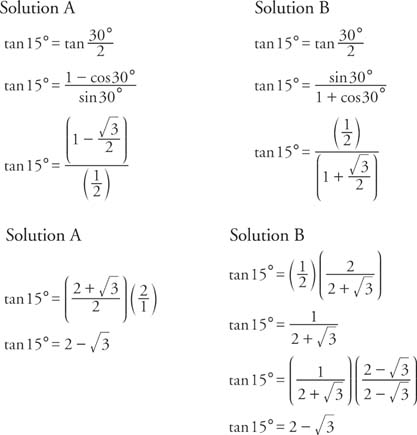## Tangent Identities

Formulas for the tangent function can be derived from similar formulas involving the sine and cosine. The sum identity for tangent is derived as follows:To determine the difference identity for tangent, use the fact that tan(−β) = −tanβ.Example 1: Find the exact value of tan 75°.

Because 75° = 45° + 30°Example 2: Verify that tan (180° − x) = −tan x.Example 3: Verify that tan (180° + x) = tan x.Example 4: Verify that tan (360° − x) = − tan x.The preceding three examples verify three formulas known as the reduction identities for tangent. These reduction formulas are useful in rewriting tangents of angles that are larger than 90° as functions of acute angles.

The double‐angle identity for tangent is obtained by using the sum identity for tangent.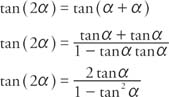The half‐angle identity for tangent can be written in three different forms.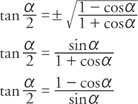In the first form, the sign is determined by the quadrant in which the angle α/2 is located.

Example 5: Verify the identity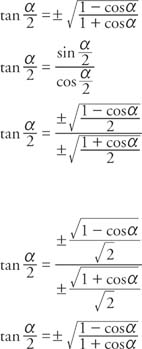Example 6: Verify the identity tan (α/2) = (1 − cos α)/sin α.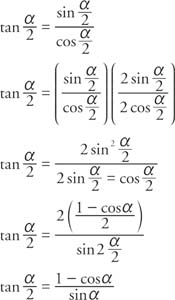Example 7: Verify the identity tan (α − 2) = sin π/(1 + cos α).

Begin with the identity in Example 6.Example 8: Use a half‐angle identity for the tangent to find the exact value for tan 15°.

What follows are two alternative solutions.